• 0

Bessel’s Correction

Bessel’s Correction

Bessel's Correction is a correction applied while calculating the sample variance and sample standard deviation where the denominator is (N-1) instead of N, where N is the sample size or the number of observations in the sample. This is done in order to correct the bias in the estimation of population variance (and standard deviations).

An application-oriented question on the topic along with responses can be seen below. The best answer was provided by Natwar Lal on 13th August 2019.

Applause for the respondents- Sreyash Sangam, Sairam Bhoopalam, Ram Rajagopalan, Mohamed Asif, Natwar Lal & Bhavesh Wakde

Also review the answer provided by Mr Venugopal R, Benchmark Six Sigma's in-house expert.

Question

Q﻿﻿. 184  Explain Bessel's correction as applies to Standard Deviation for a sample.

Note for website visitors - Two questions are asked every week on this platform. One on Tuesday and the other on Friday.

Recommended Posts

• 0

What is Bessel's Correction? - It corrects the bias in the sample variance and standard deviation where the denominator is changed from N to N-1.

Why is it required? - It is required because it is difficult to determine the population variance and standard deviation.

We all know, that due to the constraints of time and money, we prefer to work with samples and not populations. Once we have the sample, we apply Descriptive Statistics to get the sample statistics. These sample statistics are then used to make inferences about the Population Parameters (which happens to be our interest area).

Assume, that we need to determine the average and variance in weight of the Indian male. Here the average weight and variance in weight are the Population Parameters. And it is also obvious that we will not be able to get these numbers by considering the entire male population of India. Hence we revert to doing sampling.

For example sake, let us assume that the average weight of the entire male population in India is 70 Kg. BUT we do not know it. Instead we need to determine it. So we picked a sample with a sample size of 10 and measured the weights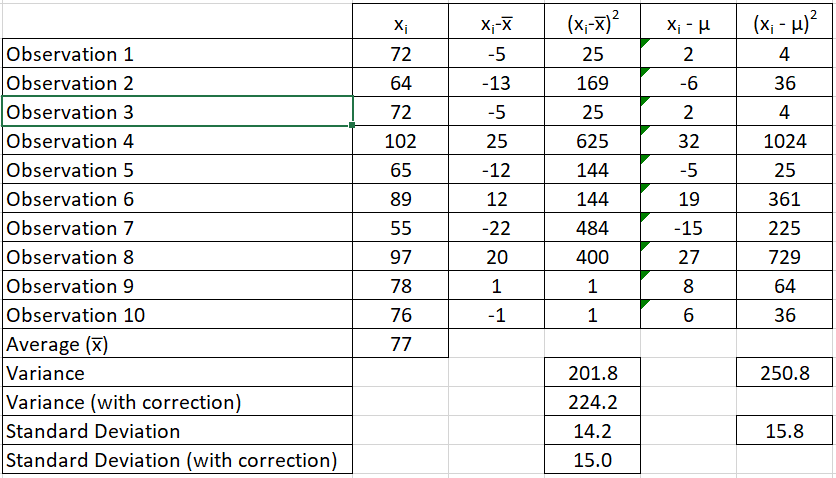The sample average is 77 Kg. This is the sample statistic that is then assumed to be the population parameter. Therefore, one would assume that the population average weight is 77 Kg (instead of the actual 70 Kg).

Now, we need to estimate the population variance and standard deviation as well (remember weight is a continuous metric and hence we also need to determine the spread in the data).

Now in column 4 (in my example), I am working with the sample average of 77 kg and then computing the variance and standard deviations (variance = 201.8 and standard deviation = 14.2). While in column 6, I worked with the population standard deviation of 70 Kg (variance = 250.8 and standard deviation = 15.8). Ideally if the population mean was known, one should be working with 70 Kg (or column 6) but since it is unknown, one could only estimate it using the sample data and sample mean.

You will notice that the variance in column 4 (201.8) is less than variance as computed in column 6 (250.8). This highlights two important facts

1. The difference is the bias

2. This bias will always make the variance or standard deviation less than what it should be if population mean is considered

I have also calculated the variance and standard deviation with Bessel correction where (N-1) or 9 is used in the denominator (variance = 224.2 and standard deviation = 15.0). You will notice that the bias is corrected to some extent. This happens as the denominator is decreased, the overall value increases.

This is Bessel's correction which is applied when population variance and/or standard deviation is to be estimated from sample mean. Bessel correction is to applied only when population mean is unknown.

Another way of understanding the Bessel Correction is by the concept of 'Degrees of Freedom'. In my example, I had a sample size of 10. Now if I pick another sample and want to keep the sample mean same, then I have the freedom to change only 9 values. I will need to keep one value fixed. This fixed value is the pivot around which the other observations can change. The same concept is applied to a population. In order to keep the population mean same while picking multiple samples, one would need to keep at least 1 value fixed. Therefore if the population size is N, the degree of freedom becomes N-1. This same N-1 is used in the Bessel Correction.

Share on other sites

• 1

Benchmark Six Sigma Expert View by Venugopal R

As most of you would have gathered, Bessel’s correction refers to using n-1 while computing standard deviation from a sample. The answer to this question can lead to an in-depth statistical discussion. However, let me try to make it simpler by elucidating the below two points of view. It is assumed that the readers are familiar with the equation used to compute standard deviation and the term ‘degrees of freedom’. Readers are also advised to do more thinking and work out some examples to gain more clarity.

Whenever we take samples from a large population, the sample means can vary, whereas there can be only one population mean. Many times, the population mean will not be known, and hence we have to use the sample mean from each sample value (xi – xbar) in the calculation for sample standard deviation. You can observe that if you substitute the sample mean (xbar) with any other value, you will get a larger standard deviation. So, assuming we know the population standard deviation and we substitute it for the sample mean, we would be getting a larger value for the standard deviation, (unless of course the population mean happens to be exactly equal to the sample mean!) This implies that the sample standard deviation calculated using the sample mean will always be lower than the true standard deviation based on population mean. We may correct this bias by reducing the numerator in the equation for standard deviation; Bessel’s correction reduces the denominator by using n-1 instead of n.

Another related aspect is about the ‘degree of freedom’. When we have ‘n’ samples, for a given mean value, except for one sample, all others have the freedom to assume different values and still achieve the same mean. In other words, n-1 samples have the freedom to vary and hence the degrees of freedom is n-1. When we calculate the ‘expected’ value of the standard deviation, we need to divide the summation of the square of differences from mean by n-1, instead of n.

When the sample size becomes larger, the difference in the standard deviations computed based on n and n-1 will narrow down.

The Bessel’s correction is applied to reduce the bias on the standard deviation calculation for a sample.

Share on other sites

• 0

Bessel's correction:

When we intend to perform the statistical analysis of data and get valuable inferences from it, there are various statistical calculations we perform which includes, Mean, Standard Deviation, Variance etc. While carrying out these statistical analysis, we have either sample of the data from a large population or the population itself.

For answering the above question lets consider the standard deviation as an example for better clarity.

If the consideration in point is the population, then the chances of data we obtain is normally accurate. This is because entire set of data from the large population is considered and hence all kind of variability is accommodated and the analysis which we obtain is  expected to be accurate, considering given conditions to be true.

However the difficulty arises when the sample size, n is small which is extracted from the BIG population. In this case, the probability of getting the exact & accurate result reduces in proportion to the reduction in sample size. In this case, the accuracy and exactness of result from the statistical data reduces. Therefore, in order provide some degree of freedom and make the calculation more accurate, one of the correction factor is incorporated which is called as Bessel's correction

In normal scenario, we consider, n as the sample size and accordingly, mean, standard deviation and variance is calculated. But in case of the Bessel's correction, n is replaced by n-1, i.e., the sample size is reduced by 1. This is turn widens the degree of freedom and increases the probability for giving more accurate/exact analysis, though not in consonance with the case when we use Population Size in overall calculation.

However, it is to be noted that, there is no standard procedure or threshold criteria when Bessel's correction has to be used. However the project leader or six sigma experts need to make decision based on the sample size of the population. When the sample size is small it is always significant to apply Bessel's correction. However if the sample size is large, than it should only be used in case of criticality of the decision making.

The unbiased method of calculating the sample variance can be calculated as below (n is being replaced with n-1):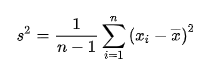Share on other sites

• 0

Usually, when you calculate any parameters on an entire population you tend to get more accurate results. However, when you working on a sample from the population you are working just with a portion of the population and, your std deviation or variance cannot be accurate.

Hence, Bessel’s correction i.e., n-1 helps in getting more accurate answers when you involve a sample.

Share on other sites

• 0

Bessel correction in statistics is used to correct the bias in the estimation of the population standard deviation. Variance calculated from sample is usually smaller, when used to estimate for population. Adjustment needs to be done to account for this.

To give a context, we can calculate the mean and variance of all students in say class 10. Now if we randomly pick a small sample of 3-4 students and calculate their standard deviation, it may not capture extremes. It is more likely to get more of the same type of students.  This standard deviation will likely be  smaller than the population. Adjustment needs to be done for the bias

Normally Variance is calculated as mean of the sum of squares of deviation of sample values from sample mean. Standard deviation is calculated as square root of the variance.

When the population mean is unknown, to estimate the population variation, (n-1) is used instead of (n) in the denominator.

This method is used when estimating population mean and variance from sample. The logic is that 1 degree of freedom is used in the sample mean and hence only (n-1) for the sample variance. When (n-1) is used, the variance will increase.

References for more mathematical reasoning and formulas:

Share on other sites

• 0

Bessel’s work includes:
correction to seconds pendulum
corrected observation of personal equation
correcting effects of instrumental errors
Bessel functions (Cylinder function)
Measuring stellar parallax and many more...…...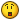Application of Bessel’s correction in Statistics, includes corrections in sample variance and sample standard deviation calculation. Correction is on the formula to use (n-1) instead of n;
where n is number of observations (in sample)

Sample Variance/Standard Deviation gives biased estimate of Population Variance/Standard Deviation. Minor Bias!

Bessel's correction is to eliminate this minor bias in sample standard deviation and sample variance calculations. So, as we use n-1, accuracy in these calculations increase significantly.

Let’s refresh standard deviation and variance calculation and deep dive with an example,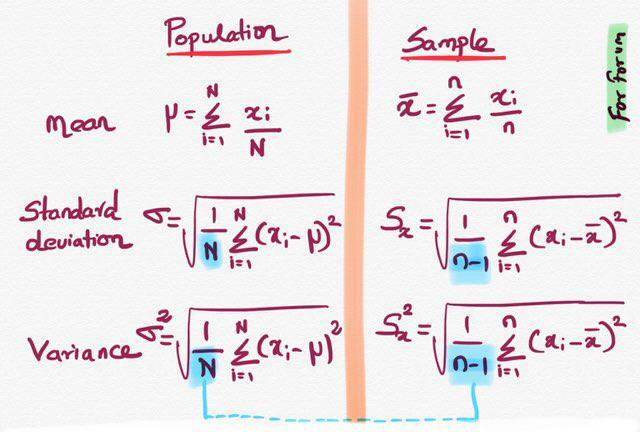Both for Standard Deviation and Variance for sample data, N is replaced with (n-1).

For Population we considered N and for sample it is (n-1)

In order to get more clarity, lets consider data set of 100 observations (Population) and

sample data randomly picked @ 5% from population data.

Quote

"The analysis of variance is not a mathematical theorem, but rather a convenient method of arranging the arithmetic." -

Ronald Fisher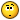For the same sample data, excel throws 2 different values,

"STDEV.P" Formula -> Considering N Samples

"STDEV.S" Formula -> Considering (n-1) during calculation

R Code:

Considering same sample Vector using R function:

sample_data <- c(82, 31, 95, 33, 92)

> mean(sample_data)
 67

> var(sample_data)
 1021.30

Verification:

> sum(sample_data)/length(sample_data)
 67

> sum((sample_Data - 67)**2)/length(sample_data)
 817.04

Manual data is different from direct calculation.
This is because, R directly applies Bessel's correction during calculation

Applying correction to Manual calculation:

> sum((sample_Data - 67)**2)/(length(sample_data)-1)
 1021.30

As the data sets are not same,

As the Samples are representations of Population

Variations Exists.... This is referred as "Sampling Variation

SAMPLES can Vary

POPULATION Is Fixed

Usually for Normal Distributed data, when we do sampling, we most likely select samples around mean and miss out lower and upper extreme values.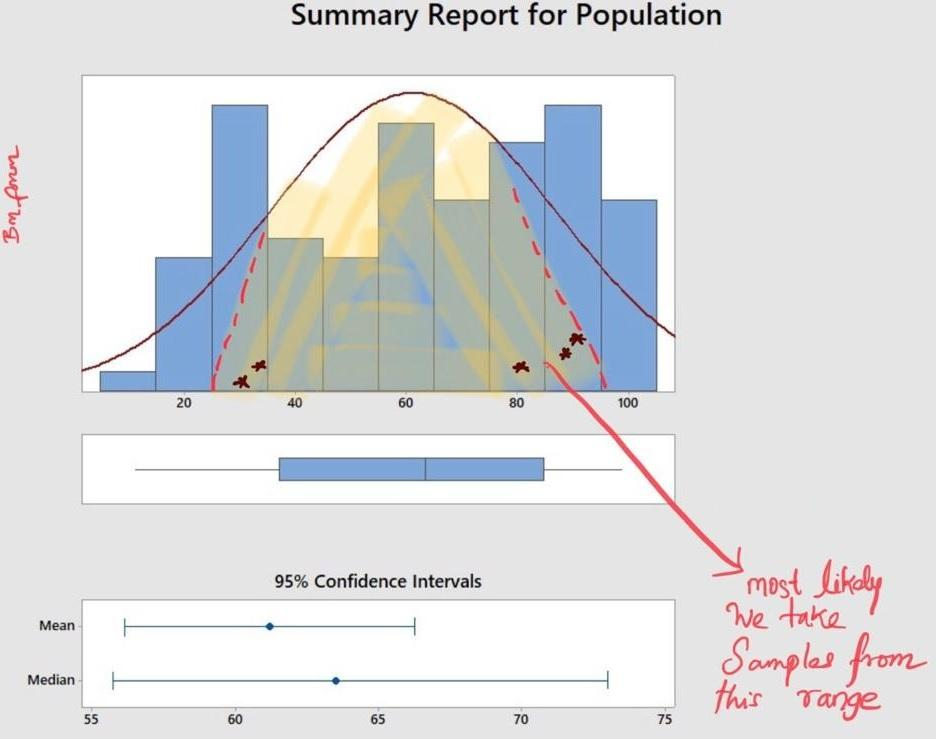Why this Bias?

This downward Bias is corrected further,

by dividing SAMPLE numerator by (n-1)

and divide POPULATION numerator by N

This is simple because of considering DoF (Degrees of Freedom)

which means Sample data loses an (1) observation [for 6 samples, DoF is 5; for 5 samples DoF is 4; for n samples DoF is (n-1)]

Dividing by (n-1) results in deriving unbiased variation.

That is, diving by DoF (Degrees of Freedom) against the Sample Size.

Less Biased Estimator and thus moving towards more accurate calculations.

Share on other sites

• 0

Variance calculation is used to find out dispersion of population(N) which contents entire set of data. This can have multiple sub set of data which can be represented by sample size(n).

Formula for the population of variance :

σ2 = Σ ( Xi - μ )2 / N

N = Population

σ2 =population variance

X     =Set of data which can range from i=1 to the population(N)

μ  = mean of population

Formula for the sample of variance is similar but  it can be categorized as biased and unbiased :

 Biased Unbiased s2n = Σ ( xi -  x_bar  ) 2 / n n = Sample size of the Population s2 = Sample variance x    =Set of data which can range from i=1 to the sample (n) x_bar = mean of sample s2n-1 = Σ ( xi -  x_bar  ) 2 / n-1 n = Sample size of the Population s2 = Sample variance x    =Set of data which can range from i=1 to the sample (n) x_bar = mean of sample

If sample drawn data are evenly getting picked up from population then its mean will be very much close to true population mean, whereas sample data drawn are picked up from particular zone there are high chance of sample mean will be far away from population mean such cases leads to underestimation to the true variance of the population. This leads to sample mean will seats between the sample data but far away from population mean which also indicates that gap between sample variance to the population variance. This phenomenon is known as biased.

To overcome this issue unbiased variance formula is getting used to find out the sample variance . This also provides the high liberty of degree of freedom during sample data collection which is getting restricted during biased variance calculation.

Share on other sites

• 0

The chosen best answer is of Natwar Lal for clearly explaining and highlighting the need for Bessel's Correction using a data set.

Also go through the answer provided by Mr. Venugopal - Benchmark's in-house expert.

Share on other sitesThis topic is now closed to further replies.
• Who's Online (See full list)

• There are no registered users currently online
• Forum Statistics

• Total Topics
3k
• Total Posts
15.1k
• Member Statistics

• Total Members
53,957
• Most Online
888